# Russian Code Cup 2012: a detailed analysis of the tasks from the final in pictures, videos and examplesOn September 10, 2012, the Russian Code Cup 2012 programming championship ended. A detailed story about how everything happened was published earlier , and today we will analyze the tasks that were proposed to the finalists. There were only six of them, and each of them is a separate interesting story:

Three hours were allocated to solve these problems. The only one to solve five of the six problems was the winner of the Russian Code Cup 2012 Vladislav Epifanov . Slightly less than half of the finalists completed four tasks each. The first three tasks did almost everything. Only one Evgeny Kapun correctly solved the problem about the deck of cards . The second place in the tournament was taken by Natalia Bondarenko , who solved four problems faster than others and with fewer attempts.

# Angry Birds

Andrey KALININ , Head of the Mail.Ru Search Developer Unit , will tell you about the problem statement .

The task is called "Angry Birds". Its essence is simple. There is a wire of finite length on which the birds are located. Some birds go to the right, some to the left. When they meet each other - they change direction. When they reach the end of the wire, they take off. It is necessary to determine when each of these birds will fly. According to the conditions of the problem, there can be up to 100,000 birds going in each direction, therefore, in total there are no more than 200,000 of them.Detailed statement of the problem
As you know, birds love to sit on wires. In the county town K there is one long wire that he especially liked. At one point, several birds were sitting on it. Everything would be fine, but only a pig running under the wire raised such a cloud of dust that it made the birds very angry.

Having become angry, the birds began to run along this wire in different directions - someone to the left, someone to the right. In this case, all birds began to run at the same speed equal to one meter per minute. When two birds meet moving towards each other, they immediately turn around and start to run at the same speed in the opposite direction.

This process would continue indefinitely, but only the wire turned out to be final, and as soon as one of the birds reaches the end of the wire, it immediately takes off, and all the other birds, stunned by this, turn around and start to run in the opposite direction. If two birds run to the edge at the same time, then two turns occur, or, what is the same, nothing happens.

You need to write a program that, according to the length of the wire, the initial positions and directions of the birds running, will find out for each bird at what point in time it will fly off the wire.

Input format

The first line contains a single integer L (1 ≤ L ≤ 109) - the length of the wire in meters.

The second line contains the number n (0 ≤ n ≤ 100 000) - the number of birds running to the right. The third line contains n different integers a i (0 <a i <L) - the distance in meters from the left end of the wire to the birds running to the right.

The fourth line contains the number m (0 ≤ m ≤ 100 000) - the number of birds running to the left. The fifth line contains m different integers b i (0 <b i <L) - the distance in meters from the left end of the wire to the birds running to the left.
No two birds are originally in the same place. It is guaranteed that at least one bird sits on the wire.

Output Format

On the first line print n integers t i- after how many minutes the ith bird running to the right will fly away in order of description in the input. In the second line print m integers u i - after how many minutes the i-th bird in the input, flying to the left, will fly away in the order of description.

Time limit - 2 seconds;

Memory limit  - 256 megabytes.

An example was given in the problem. To clarify its formulation and solution, it will be useful to track the movement of birds every minute on this example. It provides the following input: two birds are on the 8th and 9th meter and go to the right, three more birds are on the 2nd, 5th and 7th meter and go to the left. The length of the wire is 10 meters. This is the initial situation. It is necessary to calculate when a bird will fly.As you can see, in the first and fifth minutes the birds fly away from the right end, in the tenth two birds fly at once, and in the thirteenth the last one flies away.

It is important to note here that the order of birds never changes. Also note that, despite the zigzags described by the birds, the number of birds moving left or right does not depend on their collision, and the nature of the change in the coordinates of movement always remains linear. This is clearly visible in the interval from the first to the fifth minute, when there were already three collisions.

On the right in two columns I indicated the positions of the birds. It immediately becomes obvious that the moment of the first take-off from the wire can be predicted - the number of minutes (= the number of meters) to the end of the wire from the bird closest to one of the ends.

It is also seen that after take-off, all coordinates tied to the movement to the right become coordinates tied to the movement to the left, and vice versa.

As a result, solving the problem reduces to maintaining two decks — data structures of the “double-ended queue” type, in which the removal of the first or last element is carried out in O (1). When a bird takes off, the extreme element is removed from the deck, and the decks themselves are swapped. Keeping pairs <Dec; how much you need to add to the numbers in it> you can find in O (1) how long and in which direction the next bird will fly away. Since the order of the birds does not change, you should additionally store a sorted array of coordinates of the original location of the birds in order to determine which of the birds left the wire.

The most beautiful solution is the one in Python3:

from collections import deque
length = int(input())
n = int(input())
right = deque(sorted(map(int, input().split())))
m = int(input())
left = deque(sorted(map(int, input().split())))
INF = 2*10**9
ans = 0
while right or left:
L = left + addLeft if left else INF
R = length - (right[-1] + addRight) if right else INF
m = min(L, R)
ans += m
if L < R:
left.popleft()
elif L == R:
left.popleft()
right.pop()
else:
right.pop()
print (ans)


The task with the birds was first made by Mikhail KEVER by the 22nd minute from the beginning of the round. After a few seconds, Vasil BILETSKY passed the task .

In total, 40 people coped with the task, 11 people started solving it. The average time to solve it is 30 minutes. Of these, the longest time to resolve is 44 minutes (not counting situations where no decisions were sent at all). All 11 people who successfully completed the first task of the first solved at least two more. With the exception of 2 cases out of 11, they all went to task B, and then to task C, that is, they completed the tasks in alphabetical order. This task is in third place among all the tasks with which the contest began.

# Trisolians

Ilya VAYSMAN , Technical Director of Allods Online, tells about the Trisolians task :

By the condition of the problem, some inhabitants of the planet Trisol indicate their age not as a single integer, like we humans, but as a vector of k integers. In a newborn Trisolian, the age vector consists of one zeros, but with each year of growing up, a certain positive number is added to each element of the age vector. The Trisolian life story is a set of all age vectors that he had throughout his life. It is well known that Trisolians with the same story do not exist. Scientists are interested in the maximum number of Trisolians whose life story ends on a vector whose sum of elements is n. You need to write a program that calculates this value modulo a prime number 7340033 = 7 * 2 ^ 20 + 1.

For example, for k = 2 there can be 8 Trisolians, whose life story ends on a vector with the sum of 5.

1. (0, 0) - (1, 1) - (2, 3);
2. (0, 0) - (1, 1) - (3, 2);
3. (0, 0) - (1, 2) - (2, 3);
4. (0, 0) - (1, 4);
5. (0, 0) - (2, 1) - (3, 2);
6. (0, 0) - (2, 3);
7. (0, 0) - (3, 2);
8. (0, 0) - (4, 1).

Detailed statement of the problem
Recently it became known that the inhabitants of the planet Trisol indicate their age not as a single integer, as we humans, but as a vector of k integers. It is believed that in a newborn Trisolian, a vector of his age consists of k zeros. As they grow older, every year, a positive number is added to each element of the age vector.

The Trisolian life story is the set of all vectors of his age that he had during his life. Earth scientists have established that there are no two Trisolians with the same story.

Now scientists are interested in what is the maximum number of Trisolians whose life story ends on a vector whose sum of elements is n. Write a program that calculates this value modulo a prime number 7340033 = 7 · 220 + 1.

Input format

The first line contains two integers n and k - the sum of the elements of the last age vector, and the number of elements in the vector (1 ≤ n ≤ 4239, 1 ≤ k ≤ 10 9 ).

Output format

Output the maximum number of Trisolians, whose life story may end on a vector whose sum of elements is n. The answer should be deduced modulo 7340033 = 7 · 2 20 + 1.A little historical background, without which the solution to the problem is probably impossible. Trisolians are residents of the planet Trisol, located deep inside the Forbidden Zone in the Galaxy of Horror. They are 100% liquid. Able to easily change their shape. Ordinary people are peaceful, which cannot be said about the ruling elite. From time to time they drink each other, moving in this way along the career ladder.

The task is relatively simple, because all its solution comes down to combinatorics. On the other hand, it immediately becomes not so simple as it seems, if you look closely at the possible range of k - the dimension of the vector (1 ≤ k ≤ 10000000000).

 N = 2 N = 3 N = 4 N = 5 N = 6 (0,0) - (1,1) (0,0) - (1,2) (0,0) - (2,1) (0,0) - (1,1) - (2,2) (0,0) - (2,2) (0,0) - (3,1) (0,0) - (1,3) (0, 0) - (1, 1) - (2, 3); (0, 0) - (1, 1) - (3, 2); (0, 0) - (1, 2) - (2, 3); (0, 0) - (2, 1) - (3, 2); (0, 0) - (2, 3); (0, 0) - (3, 2); (0, 0) - (4, 1). (0, 0) - (1, 4); (0,0) - (1,1) - (2,2) - (3,3) (0,0) - (1,1) - (4,2) (0,0) - (1,1 ) - (2,4) (0,0) - (1,1) - (3,3) (0,0) - (1,2) - (3,3) (0,0) - (1, 2) - (2,4) (0,0) - (2,1) - (3,3) (0,0) - (2,1) - (4,2) (0,0) - (2 , 2) - (3.3) (0,0) - (3,1) - (4,2) (0,0) - (1,3) - (2,4) (0,0) - ( 5.1) (0.0) - (1.5) (0.0) - (4.2) (0.0) - (2.4) (0.0) - (3.3) 1 option 2 options 4 options 8 options 16 options

The key idea for understanding the solution is that the "bushes" for large values ​​of N partially consist of solutions for lower values, which allows us to organize calculations through recursion.

Graphically, for the special case k = 2, the life story can be displayed as a set of segments connecting the zero mark with positive integer marks lying on the axis on the line f (x) = nx.

Here's what the solution for N = 4 looks like: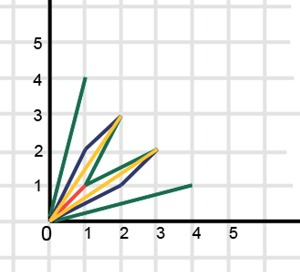For N = 4 stories, eight.

Now add vector (1,1) to this story and get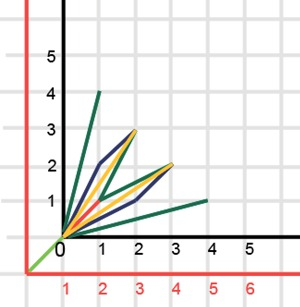Each of the stories starting with vector (1,1) will be part of the story for N = 7. Another part of the story will be smaller “bushes” starting with vector (1,2), etc.

A similar story with other vectors. We give an example for the vector (1,3), which is the beginning of a part of the elements of history for N = 7. In this case, the following will come out: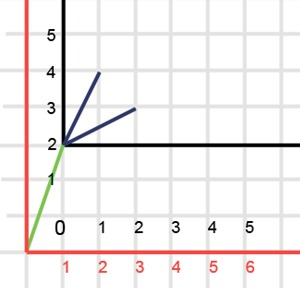That is, if we take the vector that was given to the Trisolian for his first year of birth and subtract it from all the vectors of the Trisolian life history, then after throwing this vector out, we actually get some life history of the Trisolian, in which the sum of the elements of the last of the vector is n - (the sum of the elements of the ejected vector).

Thus, we can understand that all stories for which the sum of the elements of the last vector is n can be obtained from the stories with the last vector having the sum of elements m for some m <n, adding some vector of positive elements with the sum equal to n - m to the whole story of life.

How many vectors with a sum equal to nm will be? The minimum vector includes one in each coordinate, therefore, all vectors, all components of k, and as a result, the sum nmk comes out. Now we need to give out units to k coordinates. The number of ways to do this is the number of combinations with repetitions. The number of combinations with repetitions is calculated as

A; the number of combinations without repetitions is calculated as

From which it is easily deduced that the number of combinations with repetitions a through b is equal to the number of combinations without repetitions a + b - 1 by b.

So in our case the number of ways is equal to C from n - m - k + k - 1 in
k, that is, C from n - m - 1 in k.

Denoting the desired value as ans n , we obtain the following recurrence formula :. It is assumed that ans 0= 1. The running time of the algorithm is O (n2).

where C (x, y) is the number of combinations of the second argument in the first, calculated as x! / (xy)! / y!

The task of Trisolians was done by almost all of those present at the final (except for two people). It took about 18 minutes to solve the problem. The first to do it was Roman RIZVANOV in 11 minutes, with a small margin - Evgeny KAPUN .

# CPU

Alexander GORNY , CIO Mail.Ru Group, will tell about the processor problem :

The task involves a certain dual-core processor that can execute 26 different instructions. Each instruction is symbolically written in the letter of the English alphabet. Programs consist of a set of instructions. It is necessary to calculate what minimum time the processor will need to process the indicated 2n programs (in turn, consisting of the specified sequence of specific instructions), if it is known that due to the architecture peculiarity, only the same instructions can be executed in two cores at a time, and n - the number of such processors.

Illustration of how a processor can process two programs at the same time: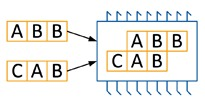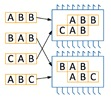The task is to efficiently scatter 2n programs on n processors and two cores in each so that the number of steps is minimal.

Detailed statement of the problem
As you know, most of the processors currently manufactured are multi-core, that is, they support the execution of several instructions simultaneously. Paraltel has developed a new type of dual-core processor that allows you to follow 26 different instructions, denoted in capital Latin letters. The execution of each such instruction requires exactly one clock cycle of the processor.

The program for this processor is a sequence of instructions. The program instructions must be executed in the order in which they follow the program; it is not allowed to interchange the instructions.

Due to the presence of two cores, the processor can simultaneously execute two programs - one on each core. However, due to architectural features, only the same instructions can be executed simultaneously on two cores of the same processor.

When two programs are executed on the processor, a special control device optimizes the execution in such a way as to complete the execution of both programs as early as possible. For example, the “ABB” and “ABC” programs can be executed on the processor in 4 cycles: first, the “A” commands of both programs on different cores are executed simultaneously, then the “B” commands at the same time, then the “B” command from the first program and, finally, "C" from the second. Similarly, the “CAB” and “BAB” programs run in 4 cycles.

Recently, company experts have assembled a supercomputer from n processors on which 2n programs are required. The organization of the calculations is such that each processor must execute exactly two programs from this set, one on each core.

You need to plan the execution of 2n programs on n processors so that the completion time for all programs is minimal.

Input format

The first line contains the number n (1 ≤ n ≤ 10) - the number of processors. Next, in 2n lines, the programs to be executed are specified. Each program contains from 1 to 100 teams. Each command is specified in a capital Latin letter.

Output format

Output a single number - the minimum time for which all programs can be executed.

As an example, a set of the following programs is given to the task: ABB BAB CAB ABC
and information that there are two processors.

These four steps are needed to run the program on two processors:

 CPU 1 core 1 CPU 1 core 2 CPU 2 core 1 CPU 2 core 2 [A] BB [A] BC [B] AB A [B] B A [B] C [C] AB AB [B] B [A] B C [A] B AB [C] BA [B] CA [B]

We divide the solution of the problem into two stages and consider each separately.

The first step is to build a graph. Its vertices are 2n programs that we need to execute. The graph will be weighted, and the weight of the edge between the two programs is equal to the amount of time that the processor spends on their joint execution. This value is equal to the sum of the program lengths minus those commands that will be executed simultaneously. And there are no more such commands than LCS (a, b), where a and b are the lines describing the programs, and LCS ( Longest Common Subsequence , the largest common subsequence) is the function that finds the largest common subsequence of two sequences.

As a result, the function of finding the weight of the ribs will look like this:

int dist(String a, String b) {
int[][] d = new int[a.length() + 1][b.length() + 1];
for (int[] ar : d) { Arrays.fill(ar, 1000000000); }
d = 0;
for (int i = 0; i <= a.length(); ++i) {
for (int j = 0; j <= b.length(); ++j) {
if (i < a.length()) {
d[i + 1][j] = Math.min(d[i + 1][j], d[i][j] + 1);
}
if (j < b.length()) {
d[i][j + 1] = Math.min(d[i][j + 1], d[i][j] + 1);
}
if (i < a.length() && j < b.length() && a.charAt(i) == b.charAt(j)) {
d[i + 1][j + 1] = Math.min(d[i + 1][j + 1], d[i][j] + 1);
}
}
}
return d[a.length()][b.length()];
}


The second stage of the solution will be finding in this graph a perfect matching in which the weight of the maximum edge is minimized. One of the simplest algorithms that solve this problem and fit into the time limits is dynamic programming, but already in subsets. Let for each set of vertices of size no more than k, we know the answer. In this case, going through all such sets and adding to each all possible edges that are not still in it, we can calculate the answer for all sets of size no more than k + 2.

	int[] d = new int[1 << (2 * n)];
Arrays.fill(d, 1000000000);
d = 0;
continue;
}
int i = 0;
while ((mask & (1 << i)) != 0) {
++i;
}
for (int j = i + 1; j < 2 * n; ++j) {
if ((mask & (1 << j)) == 0) {
d[mask | (1 << i) | (1 << j)] = Math.min(d[mask | (1 << i) | (1 << j)], Math.max(d[mask], dist[i][j]));
}
}
}


The task "Processor" was done by almost all of those present at the final (except for two people). The average time to solve this problem is about 18 minutes. The first to solve the problem was Vladislav EPIFANOV in the 11th minute.

# Siege

About the task "Siege" will tell Pavel KROTKOV , a student of the department of NRU ITMO.

The task tells about the fabulous city of Aldersberg, on which hordes of enemies are about to attack. In order to keep the city, you need to put up a barrier of n artifacts, and activate each i-th artifact with magical energy in the amount of a [i] “merlins”. After that, using b [i] “Merlins”, the enemy will be able to destroy this artifact from a distance. Defenders of the city have A maglin of magical energy, in the arsenal of the enemy - B merlin. The townspeople decided to activate the artifacts so that after their destruction by the enemy magicians, the maximum number of artifacts remains active. It is necessary to help the defenders of the city choose which artifacts to activate.

Detailed statement of the problem
Hard times have come for the glorious city of Aldersberg. From minute to minute, countless hordes of enemies will go on the assault. Only magical barriers can help hold the city.

There are n artifacts in the arsenal of the city’s defenders to put up a barrier. To activate the i-th artifact, a i merlins (units of magical energy) are required . After that, using b i merlins, the enemy can destroy the artifact from a distance.

Defenders of the city have A maglin of magical energy, in the arsenal of the enemy B merlin. Magical energy reserves are not replenished. The townspeople decided to activate the artifacts so that after their destruction by the enemy magicians, the maximum number remains active.

Help the city’s defenders choose which artifacts to activate.

Input format

The first line contains three integers A, B and n (0 ≤ A, B ≤ 105, 0 ≤ n ≤ 1000), separated by spaces. The next n lines contain pairs of numbers ai and bi (1 ≤ a i , b i ≤ 105).

Output format

On the first line, print the number of artifacts that will remain active during the optimal actions of both sides of the conflict. In the second line print the number of artifacts that the defenders of the city should activate, and the numbers of these artifacts. Separate numbers on one line with spaces.

Artifacts are numbered starting from one in the order in which they are specified in the input.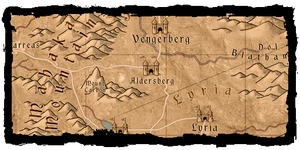A little historical background. Aldersberg is the second largest city in one of the four Northern Kingdoms, Aedirne, located on the very edge of the world. The city of Aldersberg was built around the walls of the fortress that guarded the borders of Aedirna ( Andrzej Sapkowski ).

Suppose that the city’s defenders activated a number of artifacts. The optimal strategy for their enemies would be to destroy them in order of increasing b i . Indeed, the enemy’s goal is to destroy as many artifacts as possible. It makes no sense to spend a lot of energy on an indestructible artifact, as long as there is an easy target.

Renumber the artifacts in non-decreasing order b i. Let it be known that the first artifact that the enemy cannot destroy with the optimal actions of the defenders is numbered k. We describe the actions of the defenders, based on this assumption. Several of the first k − 1 artifacts should be selected so that the total energy spent on their destruction is at least B − b k + 1 merlins, and the activation energy is minimal. Activate the k-th artifact. Finally, we activate the last n − k artifacts in order of increasing energy consumption.

Unfortunately, k is not known in advance. We will sort out the number of the first artifact that the enemy cannot destroy, and build the optimal answer for this assumption.

Let D ij- the minimum energy that will be required to activate artifacts with numbers no more than i (up to 1000), such that for their destruction j merlins of magic energy (up to 100000) will be required. Transition: D i, j = min (D i − 1 , j, D i − 1 , j − b i + a i ). These two cases correspond to whether the artifact number i must be activated in order to achieve the optimal D ij .

From the array D we find the energy necessary for the enemy to not be able to destroy the k-th artifact. After that, we eagerly take the rest, while there is enough energy to activate. As a result, going through all k, we find the optimal answer.

The running time of the described approach is O (n (B + n)). In order to turn it into a complete solution, you need to add information storage to restore the answer, which does not affect the asymptotic behavior.

The bottleneck in the solution could be the memory used. Note that the D array can only store the last row. In addition, for each pair (i, j) it is enough to know whether it is necessary to activate artifact number i in order to achieve the optimal D ij . In order to meet the memory limits, it was necessary to use no more than two bytes to store this bit of information.

The siege task was solved by 19 people. Peter MITRICHEV completed the task faster than anyone in the 87th minute (before the Siege task, Peter successfully passed the first three).

# Shuffle deck

Sergey MELNIKOV , student of NRU ITMO, talks about the task of mixing the deck :

In this problem, you need to think over the deck mixing mechanism, which allows you to mix it perfectly (for the minimum number of moves of the card blocks from the middle to the end of the deck (that is, make sure that no two identical cards go one after the other), or report that it is impossible to mix the deck perfectly . Initially, the deck is sorted by non-decreasing dignity. Given the number of different advantages of the cards, the order of the cards in the deck, the number of decks.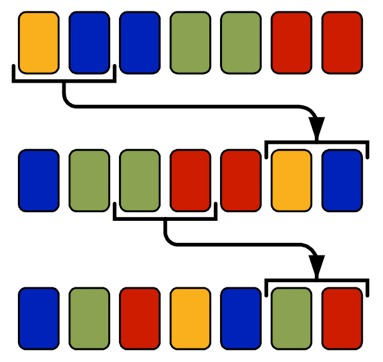Detailed statement of the problem
Automation and computerization are rapidly invading all areas of our lives. Dishwashers, on-board computers in cars, electric fly swatter - all these devices to one degree or another facilitate the person’s standard habitual actions. In the same task, you will be required to automate another action familiar to many - shuffling a deck of cards.

Consider a new deck of cards, the cards in which have some characteristic, which is an integer from 1 to t. We will call it card value, several cards in a deck can have the same value.

Initially, the cards in the deck are sorted by non-decreasing value. Accordingly, the first card in the deck has a value of 1, and the last - a value of t. Moreover, the merits of two adjacent cards in the deck differ from each other by no more than one, and the merits of each next card are no less than the merits of the previous one.

We will call a deck well mixed if no two consecutive cards have the same value.

To mix the deck, a device is used that is capable of performing the following types of actions: for some i and j, take cards that are in positions i-th to j-th inclusive and move them to the end of the deck in the same order.
You need to write a program that, according to the description of the original deck, will make up a sequence of the minimum number of specified actions, as a result of which the deck will be well mixed.

Input format

The first line contains a single positive integer x - the number of decks to be sorted. Next are the descriptions of x decks.

The description of each deck consists of two lines. The first line of the deck description contains a positive integer n - the number of cards in the deck. The next line contains n natural numbers describing the advantages of the cards in the deck. The first number in such a line is always equal to one, each subsequent one is not less than the previous one and differs from it by no more than 1.

The total number of cards in all the decks in the input does not exceed 200,000.

Output format

For each deck given in the input, output "-1" if it is impossible to mix it using the steps described in the condition. If the mixing algorithm exists, then in the first line of its description print k - the minimum number of actions that are required to mix the deck. In the next k lines, print the actions, each of which is described by two numbers i and j - the positions of the cards that limit the segment that needs to be moved to the end.

We will call conflict , a situation in which two identical cards stand in a row: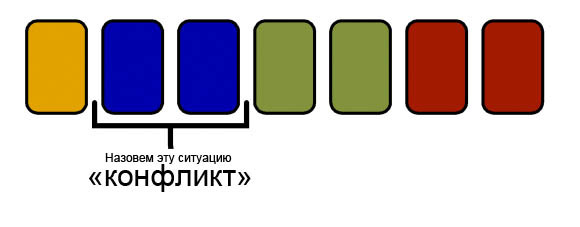We call the body the initial sequence of cards, which we will gradually reduce by transferring the cards to the tail. The tail will be formed in such a way that the cards in it do not form conflicts.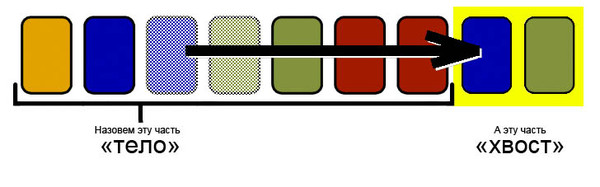We transform the original sequence into a sequence of conflicts and will work with the new sequence. We will call the color of the conflict the number written on the cards that form it. Each time transferring a segment of cards to the end of the deck, we will choose its ends inside conflicts.

We denote the number of conflicts by K. Since one move operation removes no more than two conflicts, it is impossible to solve the problem faster than in ⌈K / 2⌉ operations (here and below in the description there are constructions ⌋ ... ⌋ and ⌈ ... ⌉ - this is rounding down and up, respectively )

We denote by M the maximum number of conflicts of one color and we will call these conflicts themselves “maximum”. We learn to solve the problem in the case when M = /K / 2⌉.

I also remind you that according to the conditions of the task, the deck is sorted by non-decreasing dignity.

For example, in deck 1122333344, the number M will be 3 (three conflicts 33), the total number of conflicts - 6. In this case, M = ⌈K / 2⌉.

In this case (not an example), there are two options:

1. K — четное (как в примере). Покрасим все конфликты, предшествующие «максимальным» в цвет A, «максимальные» конфликты в цвет B, а следующие за ним — в цвет C. |A| + |B| + |C| = |K|, следовательно |A| + |C| = |B| = M. В приведенном выше примере цветовая раскраска для 1122333344 будет AABBBC.

Будем ликвидировать конфликты парами: сначала пары (B, C), потом, когда таких пар не останется — пары (A, B). Отметим, что хвост будет иметь вид (B, C)* (A, B)*, где * — одно или более повторение.

В нашем примере это:
1122333344 (AABBC) ->1122333-4.34 (AAB) -> 1-2333-4.3412 (BB)

(дефисы обозначают места, откуда взяты карты перед переносом в хвост. Точка – разделитель тела и хвоста)

Очевидно, два конфликта одного цвета подряд не идут. При сопряжении с телом тоже все будет хорошо: если |C| > 0, то последняя карточка со значением C останется на месте, а хвост будет начинаться с B. Если же |C| = 0, то хвост имеет вид (A, B)*, при этом последняя карточка со значением B останется на месте.

2. K — нечетное. Применим аналогичную раскраску. Если есть хотя бы одна карточка цвета A, то сведем задачу к предыдущему случаю, добавив в начало виртуальную карточку и A-конфликт. Если есть карточка цвета C, то добавим новую виртуальную карточку и C-конфликт в конец, опять сведя к предыдущему случаю.

The solutions for these cases are optimal, since the lower limit of the number of operations ⌈K / 2⌉ is reached. In total, we are able to optimally solve the problem when the number of “maximum” conflicts is half of all conflicts (with rounding up). We will call such a case basic.

Now we classify the initial situations by the maximum number of cards of the same value in a row. We will call these cards maximum and denote their number as Q.

• Q = 0. There are no conflicts, nothing needs to be done.
• If Q> ⌈N / 2⌉, then the problem has no solution. In this case, we have less than Q − 1 not maximum cards, which is not enough to separate cards of maximum value.
• Q = ⌈N / 2⌉. В этом случае не максимальных карточек хватает, но каждая вторая карточка должна быть максимальной. Покрасив карточки в три цвета получим:

• a A-карточек идущих до максимальных,
• b = Q B-карточек являющихся максимальными
• c C-карточек после максимальных.

При этом будем считать, что все A- и C-карточки образуют конфликты Рассмотрим следующие случаи

• a = 0. Следовательно c = N − Q = ⌊N / 2⌋, и, следовательно, b отличается от c не более чем на единицу, то есть мы свели к базовому случаю.
• с = 0. Аналогично предыдущему случаю.
• a > 0, b > 0 Среди А-карточек a − 1 конфликт, среди B: b − 1, среди C: c − 1. (a − 1) + (c − 1) = N − Q − 2, отличается от Q − 1 не более чем на единицу, а это значит, что это базовый случай.

• M < ⌊ ( N + 1) / 2 ⌋. Если можно добавить некоторое количество виртуальных конфликтов (конфликтов между карточками с разными значениями), чтобы задача свелась к базовому случаю, то добавим их и решим как базовый случай.

Now, each time, we will delete the last pair of conflicts that can be deleted (the last two conflicts are of different colors) (C 1 , C 2 ). If in the process we get into the base case, then we will decide, since we solve it. If there are no more conflicts left, then the problem is solved.

We prove that no new conflicts will arise. Consider the color of the last conflict Z, we will transfer pairs of conflicts (X, Z) until Z runs out, while Z remains at the end and get the tail Z (X 1 , Z) (X 2 , Z) .... If the Z-conflicts run out, then there will be a transition to (X, Y) conflicts, that is, the pair (X k , Z) (X k + 1 , Y), for X k + 1 ≠ Z.

In the transition to the base case, there will be no conflict, because after transferring the pair (X, Z), neither X nor Z can become maximal (M). Since M ≠ Z, either Z ended and the conflict (X, Z) (Z, ...) does not arise, or Z is after M, that is, we get the continuation (X, Z) (M, Z).

Since we remove a couple of conflicts at each step, or use the base case case, the constructed solution is optimal and works for ⌈K / 2⌉ operations.

You may notice that there is no need to store information about which numbers and in what order are written in the tail, since there are no conflicts. The sequence of values ​​can be maintained using the Cartesian tree with an implicit key, and the total operating time will be O (N log N). Also, if at some point we determine that there are no conflicts at the end of the body, then we can reduce the length of the body and accordingly increase the tail. You can consider all the cases when a segment moves, and make sure that the body will always look like: the prefix of the original array with no more than two cut sub-segments. Based on this fact, it is possible to implement a solution in O (n), but to solve this problem in these restrictions this is not required.

The task "Shuffling cards" was solved only by Eugene KAPUN.

# Race track

The condition of the problem is told by Nikolay VEDERNIKOV (ITMO):

According to the conditions of the problem, a new racing track was built in a certain county city N. The track is completely ready for operation, it is only necessary to register it in the World Register of Racing Tracks, but for this it is necessary to find out its length.

The track is a space bounded by the outer and inner edges of the track. We will call a simple closed polygonal line that does not have self-intersections and self-touches. Both the outer and inner edges are arbitrary simple closed broken lines, while the broken line of the inner edge is strictly inside the polygon formed by the outer edge of the trace. A trajectory of movement along the route is any simple closed polyline contained in the polygon of the outer edge of the route, while containing the inner edge of the route in the polygon formed by it.

The trajectory can have common points with both the internal and external edges of the path.

The length of the track, according to the rules of the World Register of Racing Tracks, is the length of the shortest path of movement along the track.

It is necessary to write a program that, according to the description of the internal and external edges of the route, will find the length of the route.

The number of links of the broken outer and inner edges of the path can be from 3 to 258 inclusive, the coordinates of the vertices are given by integers from –1000 to 1000, the broken lines do not have common points and are specified in counter-clockwise order.

The literal statement of the problem can be found on the official website of the Russian Code Cup .

This task is about planimetry and about working with the graph of the vertices of the edges of the route.

We divide the solution of the problem into two stages and consider each separately.

The first step is to build a graph whose vertices are all the vertices of the edges of the route. Two vertices are connected by an edge, if the segment drawn between the vertices does not extend beyond the limits of the path. The graph will be weighted, and the weight of the edge between the two vertices will be equal to the distance between them.

To build a graph, it is necessary to check whether the segment leaves the limits of the trace. This can be done by crossing the segment with all links of both broken lines.

It is also worth paying special attention to the case when the segment extends beyond the limits of the track at the top of the polyline. To avoid this, we can assume that the segment, with the exception of its ends, should not have common points with the edges of the path.

Another case of an invalid segment is a segment that lies completely in the polygon of the inner edge of the path or outside the polygon of the outer edge. You can cut off such segments if you carefully consider the angles at which the segment itself and two links corresponding to this end of the polyline exit from one of the ends of the segment.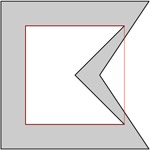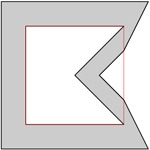The leftmost vertex of the inner edge of the path will always lie on the optimal path, and will be the left point of the optimal path. It is required to exclude from the graph all vertices to the left of this one, and to split the vertex itself. In this case, the ribs must be divided between two copies of the vertex in accordance with whether they are above or below the links of the broken inner edge. This can also be done by considering the angles at the apex.The second stage of the solution will be finding in this graph the shortest path between two copies of a divided vertex. The shortest path will be the optimal route, and its length is the length of the route. The shortest can be found using Dijkstra's algorithm.

The construction of the graph takes O (n 3 ) time, since each of the O (n 2 ) possible edges must be crossed with O (n) links of the broken line. The time to find the shortest path in a graph with O (n) vertices and O (n 2 ) edges is O (n 2 ). Thus, the total runtime of the program is O (n 3 ).

The task "Race track" was successfully solved by five people. The first of them was Vladislav EPIFANOV, he passed the task in the 93rd minute after successfully solving the first three.

These are the tasks of the final of the Russian Code Cup 2012 . In order to get to the finals, it was necessary to solve the problems proposed at one of the three qualifying online stages (they were much simpler, I published the analysis of the first , second and third qualifying rounds), and after reaching the first two hundred of the best - in the online qualifying round ( parsing ) to get into the top fifty participants. Many of the tasks formulated in a game form are analogues of the real problems that arise in the real world. Of course, we could not tell you the real name of the planet Trisol or the secret city of Aldersberg, but we are glad that we helped them, right?

Aliev Rauf
Director of Research and Education
Mail.Ru Group